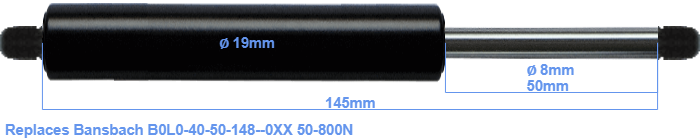# Replacement for Bansbach B0L0-40-50-148–0XX 50-800N

\$23.77

Replacement gas spring for the Bansbach B0L0-40-50-148--0XX 50-800 Newton. Thread: M8. Brand: Stabilus Industry Line.
 Force Choose an option50 Newton60 Newton80 Newton100 Newton120 Newton140 Newton150 Newton160 Newton180 Newton200 Newton220 Newton240 Newton250 Newton260 Newton280 Newton300 Newton320 Newton340 Newton350 Newton360 Newton380 Newton400 Newton420 Newton440 Newton450 Newton460 Newton480 Newton500 Newton520 Newton540 Newton550 Newton560 Newton580 Newton600 Newton620 Newton640 Newton650 Newton660 Newton680 Newton700 Newton720 Newton740 Newton750 Newton760 Newton780 Newton800 NewtonClearThe diameter of the black cylinder of this gas spring is 19 millimeter. The rod has a diameter of 8 mm. The chrome part is called the rod. It has a length of 50 mm. The total length of this gas strut is 145 mm. This is the total distance between the centers of the two mounting parts. The distance from thread to thread (without mounting parts) is 145 mm. This gas spring (B0L0-40-50-148--0XX) has a force of 50-800 Newton. This is not an original gas spring produced by Bansbach, but it can replace a Bansbach gas spring. This a gas spring with the brand Stabilus Industry Line. The force (50-800 N) and dimensions are identical to the original.